Nanomedicine, Volume I: Basic Capabilities

Robert A. Freitas Jr., Nanomedicine, Volume I: Basic Capabilities, Landes Bioscience, Georgetown, TX, 1999

10.1.2.2 Mechanical Oscillators

The most accurate macroscale mechanical clock was the Shortt pendulum clock, the primary timekeeper at the Greenwich and U.S. Naval observatories until the late 1940s. The Shortt clock consisted of a pendulum swinging freely in near-vacuum at constant temperature, driven by another identical clock slaved to the first; frequency stability was Dn / n ~ 4 x 10-8.1696

In the nanoscale realm, Drexler10 proposes a nosc ~ 1 GHz mechanical clock intended to drive a nanomechanical computer CPU (Section 10.2.1). In this clock, a DC electrostatic motor (Section 6.3.5) turns a crankshaft that converts rotary motion into sinusoidally oscillating linear motions of a drive rod. A cam surface on the drive rod forces a follower rod into up or down positions, generating regular clock pulses with intervals determined by the position of the follower with respect to the mean position of the ramp on the cam surface. Clock stability is limited by the stability of the crankshaft rotation rate. The proposed DC electrostatic motor has radius Rmotor = 195 nm, rim speed vrim = 1000 m/sec, and output power Pmotor = 1.1 microwatt, giving a developed torque of tmotor ~ 2 x 10-16 N-m. From Eqn. 4.23, the minimum detectable force (e.g., to establish feedback control, such as a Watt governor) for a 195-nm force sensor with 99% reliability is at most ~0.4 pN, giving a minimum detectable torque of tmin = 8 x 10-20 N-m. At constant torque, variation in crankshaft angular velocity Dw / w (= Dn / n) ~ tmin / tmotor = 4 x 10-4.

Numerous alternative mechanical oscillators are readily conceived:

A. Tuning Fork -- A diamondoid tuning fork with tines of length Lfork = 100 nm, half-thickness Rfork = 10 nm, density rfork = 3510 kg/m3 and Young's modulus Efork = 1.05 x 1012 N/m2 has a natural vibrational frequency of:1697,1698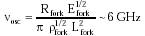{Eqn. 10.1}

In 1998, Sandia National Laboratories began offering a product line of electrostatically-driven ~1 MHz polysilicon "tuning fork" microresonators.

B. Helical Spring -- A helical spring with spring constant ks = 10 N/m and mass mspring ~ 2 x 10-19 kg (~40-nm-edge diamond cube) has a natural vibrational frequency of: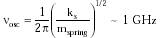{Eqn. 10.2}

ignoring gravity and spring mass.

C. Circular Membrane -- A flexible, thin circular membrane of radius Rmembrane = 100 nm, uniform density rmembrane = 3510 kg/m3, and thickness hmembrane = 10 nm, if clamped at its boundary and stretched by a tension Fmembrane (the force per unit length anywhere in the membrane), has characteristic frequencies of vibration of :1698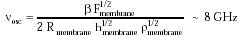{Eqn. 10.3}

where Fmembrane ~ 100 N/m for a 10-nm thick diamondoid sheet stretched to near a conservative working stress of ~1010 N/m2, and b is a constant of order unity, derived from the roots of Bessel functions for various diametral and circular vibrational nodes.

D. Torsional Pendulum -- Another oscillator is the torsional pendulum, wherein a diamondoid rod of radius Rrod = 35 nm, length Lrod = 1900 nm, density rrod = 3510 kg/m3, and shear modulus Grod = 5 x 1011 N/m2, is clamped at one end and the other end twists around the longitudinal axis in vacuo at a characteristic oscillation frequency:1164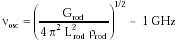{Eqn. 10.4}

If the end of this rod twists through an amplitude DX, then the time averaged power loss due to shear radiation10 is Prad = p Rrod6 DX2 Grod3/2 / 256 Lrod6 rrod1/2 ~ 26 pW for DX < 95 nm, a rod strain of DX / Lrod <~ 5% and an oscillator power density of Drod = Prad / p Rrod2 Lrod ~ 4 x 109 watts/m3. The stored torsional energy is Erod = (1/2) ktorsion q2 = p Grod DX2 Rrod2 / 4 Lrod, so the characteristic decay time for the oscillation in the exemplar system is: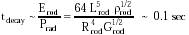{Eqn. 10.5}

giving plenty of access time for clock resonance driver mechanisms. Aside from exogenous noise sources, which can be considerable in magnitude but may be filtered out by good design, one important endogenous source of uncertainty in nosc is the elastic longitudinal displacement (DL) for the end of a thermally excited rod that randomly alters rod length, slightly changing the frequency. For bulk diamond at T ~ 300 K, and ignoring entropic contributions which become important only in longer, narrower rods than in this example, DL ~ 10-16 Lrod1/2 / 2 Rrod (Tables 5.8 and 5.16 in Drexler10). Since nosc ~ Lrod-1, then Dn / n ~ DL / Lrod and so:{Eqn.10.6}

for kelast ~ 2.5 x 10-33 m3, Lrod = 1900 nm, and Rrod = 35 nm. Another systemic source of uncertainty is rod length variation due to the slowly temporally- and spatially-varying ambient human body temperature. Given a coefficient of volume expansion bD = 3.5 x 10-6 K-1 for diamond,567 a typical variation of DT ~ 3 K per tcirc ~ 60 sec circulation time for bloodborne nanorobots, and a thermal recalibration time of trecalib ~ 1 sec (to correct for temperature dependence), then Dn / n ~ trecalib DT bD / tcirc ~ 2 x 10-7. J. Soreff notes that yet another limitation on oscillator accuracy is the 1/Q resonance width, which is a function of the design details.

Clock accuracy may be defined by a time measurement error over a single observation cycle (Nobs = 1) of Dterror = tactual - tclock, where tactual = (nosc)-1 and tclock = (nosc (1 + Dn / n))-1. Time measurement errors over multiple cycles (e.g., Nobs = nosc tobs > 1), spanning a total observation time tobs between clock recalibrations, are randomly distributed around zero but do not sum to zero, constituting instead a random walk with a maximum excursion of: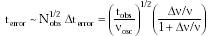{Eqn. 10.7}

This assumes that the errors are uncorrelated -- e.g., for thermal fluctuation errors, individual observation times must lie at least tEQ apart, where tEQ is the time required for thermal equilibration with the environment (Eqn. 10.24). For the exemplar oscillator with Dn / n ~ 10-6 at nosc = 1 GHz, accumulated terror ~ 1 nanosec during a continuous (uncalibrated) observation time of tobs ~ 1000 sec.

Last updated on 23 February 2003